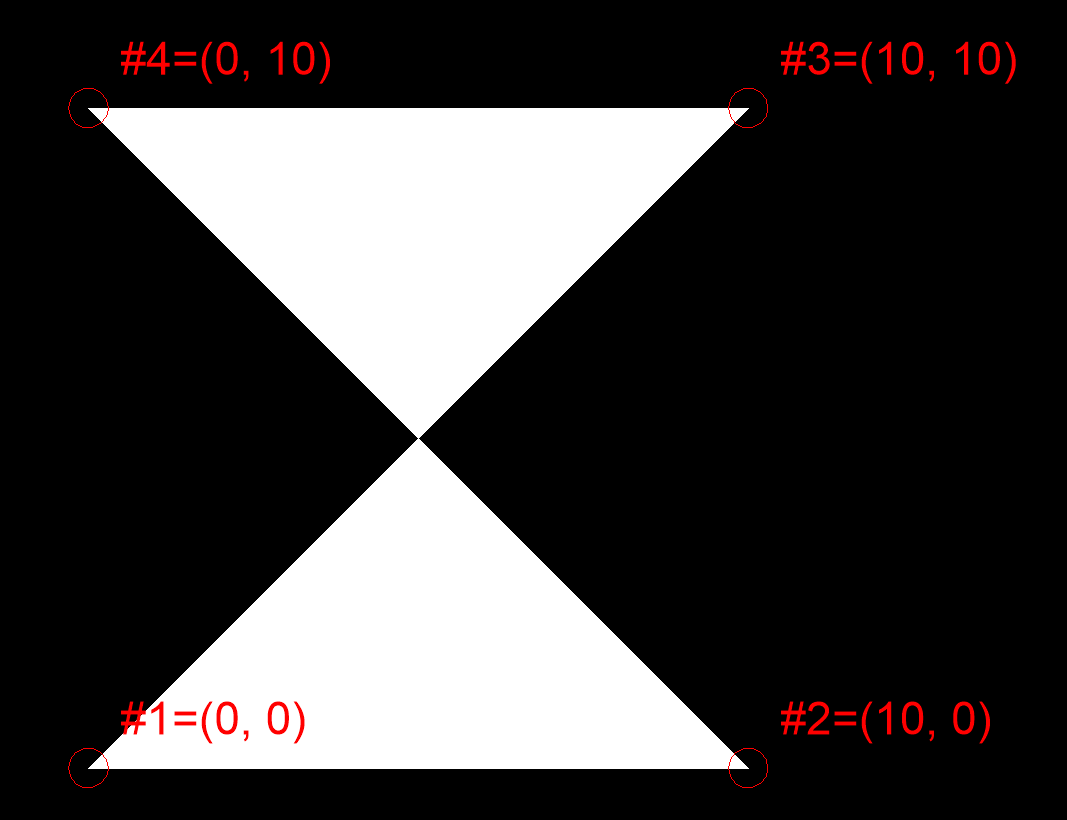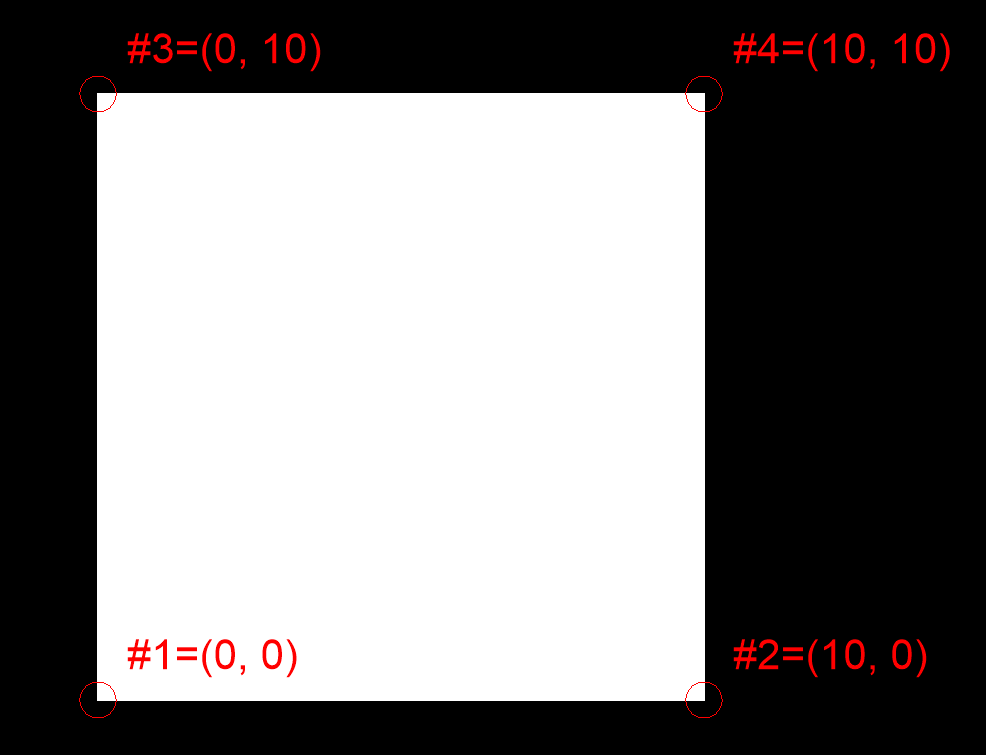# Solid¶

The SOLID entity (DXF Reference) is a filled triangle or quadrilateral. Access vertices by name (`entity.dxf.vtx0 = (1.7, 2.3)`) or by index (`entity = (1.7, 2.3)`). If only 3 vertices are provided the last (3rd) vertex will be repeated in the DXF file.

The SOLID entity stores the vertices in an unusual way, the last two vertices are reversed:

```msp.add_solid([(0, 0), (10, 0), (10, 10), (0, 10)])
```Reverse the last two vertices to get the expected square:

```msp.add_solid([(0, 0), (10, 0), (0, 10), (10, 10)])
```Note

The quirky vertex order is preserved at the lowest access level because ezdxf is intended as a DXF file format interface and presents the content of the DXF document to the package user as natively as possible.

The `Solid.vertices()` and `Solid.wcs_vertices()` methods return the vertices in the expected (reversed) order.

 Subclass of `ezdxf.entities.DXFGraphic` DXF type `'SOLID'` Factory function `ezdxf.layouts.BaseLayout.add_solid()` Inherited DXF attributes Common graphical DXF attributes

Warning

Do not instantiate entity classes by yourself - always use the provided factory functions!

class ezdxf.entities.Solid
dxf.vtx0

Location of 1. vertex (2D/3D Point in OCS)

dxf.vtx1

Location of 2. vertex (2D/3D Point in OCS)

dxf.vtx2

Location of 3. vertex (2D/3D Point in OCS)

dxf.vtx3

Location of 4. vertex (2D/3D Point in OCS)

transform(m: Matrix44) Solid

Transform the SOLID/TRACE entity by transformation matrix m inplace.

vertices(close: bool = False) list[Vec3]

Returns OCS vertices in correct order, if argument close is `True`, last vertex == first vertex. Does not return the duplicated last vertex if the entity represents a triangle.

wcs_vertices(close: bool = False) list[Vec3]

Returns WCS vertices in correct order, if argument close is `True`, last vertex == first vertex. Does not return the duplicated last vertex if the entity represents a triangle.